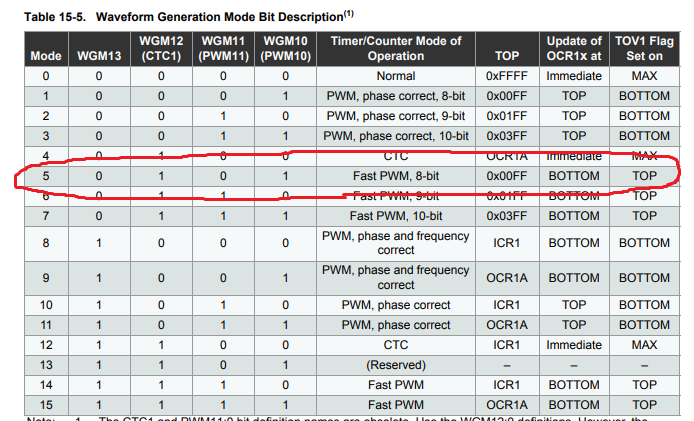## configuration OC1A and OC1B as PWM at a same time

6 posts / 0 new
Author
Message

hello friends

I need to use the OC1A and OC1B  as PWM  at same time , but when i configure OC1A and OC1B as PWM at same time only one of them generate a PWM .

Can someone help me to solve this problem?

I use ATMEGA328P ;

THIS IS MY CODE :

```// - - - DIGITAL PIN 9 PWM (PB1-OC1A) - - - //
void PWM_digPin9_init(int invert_pwm9)
{
if (invert_pwm9==1)
{
TCCR1A=(1<<WGM10) |(1<<COM1A1) |(1<<COM1A0);
}

else{
TCCR1A=(1<<WGM10) |(1<<COM1A1);
}

TCCR1B=(1<<WGM12)|(1<<CS12) ;
DDRB |= (1<<PINB1)	;

}

void PWM_digPin9_Output(uint16_t duty)
{
OCR1A=duty;
}

// - - - DIGITAL PIN 10 PWM (PB2-OC1B) - - - //

void PWM_digPin10_init(int invert_pwm10)
{
if (invert_pwm10==1)
{
TCCR1A=(1<<WGM10) |(1<<COM1B1) |(1<<COM1B0);

}

else{
TCCR1A=(1<<WGM10) |(1<<COM1B1);
}

TCCR1B=(1<<WGM12)|(1<<CS12) ;
DDRB |= (1<<PINB2)	;

}

void PWM_digPin10_Output(uint16_t duty)
{
OCR1B=duty;
}```

This topic has a solution.
Last Edited: Fri. Jan 11, 2019 - 03:09 PM

Personally I find code such as:

```if (invert_pwm9==1)
{
TCCR1A=(1<<WGM10) |(1<<COM1A1) |(1<<COM1A0);
}

else{
TCCR1A=(1<<WGM10) |(1<<COM1A1);
}```

quite confusing. The only thing changing as the result of the inversion is the COM1A0 setting so surely this would be clearer as:

```TCCR1A=(1<<WGM10) |(1<<COM1A1);
if (invert_pwm9==1)
{
TCCR1A |= (1<<COM1A0);
}```

What I was actually looking for is exactly what WGM mode it was you are selecting. Can I assume that it is in total WGM12 | WGM10 which from:which would appear to be mode 5 (Fast 8 bit PWM with TOP = 0xFF) ?

PS as to your actual problem I don't think you are showing enough code. You should show how/where PWM_digPin9_init/PWM_digPin10_init are being called. But as they seem to be "either/or" in that you either set COM1A bits or COM1B bits but with no provision to have both set then it seems fairly obvious why you can only get one channel to work.

This reply has been marked as the solution.

Surely the first step is to indent the code neatly.   Then a human (i.e. you) can read and understand it.

```// - - - DIGITAL PIN 9 PWM (PB1-OC1A) - - - //
void PWM_digPin9_init(int invert_pwm9)
{
if (invert_pwm9 == 1)
{
TCCR1A = (1 << WGM10) | (1 << COM1A1) | (1 << COM1A0);
}

else {
TCCR1A = (1 << WGM10) | (1 << COM1A1);
}

TCCR1B = (1 << WGM12) | (1 << CS12) ;
DDRB |= (1 << PINB1)    ;

}

void PWM_digPin9_Output(uint16_t duty)
{
OCR1A = duty;
}

// - - - DIGITAL PIN 10 PWM (PB2-OC1B) - - - //

void PWM_digPin10_init(int invert_pwm10)
{
if (invert_pwm10 == 1)
{
TCCR1A = (1 << WGM10) | (1 << COM1B1) | (1 << COM1B0);

}

else {
TCCR1A = (1 << WGM10) | (1 << COM1B1);
}

TCCR1B = (1 << WGM12) | (1 << CS12) ;
DDRB |= (1 << PINB2)  ;

}

void PWM_digPin10_Output(uint16_t duty)
{
OCR1B = duty;
}```

Now that you can read it,  it is easy to see that your initialisations interfere with each other.

It would be far simpler to just write a single init function e.g.

```void PWM_digPin9_10_init(int invert_pwm9, invert_pwm10)
{
uint8_t com9 = (2 << COM1A0);
uint8_t com10 = (2 << COM1B0);
if (invert_pwm9) com9 = (3 << COM1A0);
if (invert_pwm10) com10 = (3 << COM1B0);
TCCR1A = (1 << WGM10) | com9 | com10;   //PWM#5
TCCR1B = (1 << WGM12) | (4 << CS10);    //PWM#5, div256
DDRB |= (1 << PINB1) | (1 << PINB2);
}```

Personally I am happier with using numbers in multi-bit fields.   It is obvious what you want to do.

My head hurts when I have to extract all the individual bits.

Of course this goes wrong when WGM1n bits are split across registers.   Just add comment.

David.

hello David thank you so much your code worked great .... :)The actual code is fairly unimportant.

1.  format code nicely.    (it is often a single keystroke command to do it automagically)

2.  use = instead of |= when initialising

3.  make a comment when something is unusual

4.  use whatever style you feel most comfortable with

It is your code.   You will have to read it in a year's time.   You will want to understand what you have done.

Good Luck.

David.